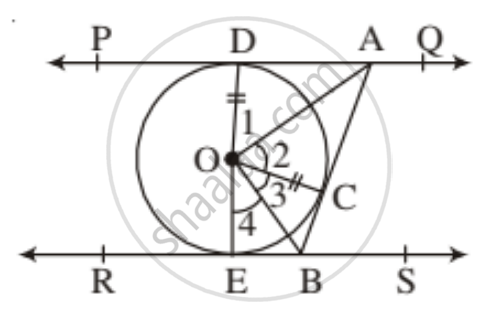# In the given figure, PQ and RS are two parallel tangents to a circle with centre O and another tangent AB with point of contact C intersects PQ at A and RS at B. Prove that ∠AOB = 90º - Mathematics

Sum

In the given figure, PQ and RS are two parallel tangents to a circle with centre O and another tangent AB with point of contact C intersects PQ at A and RS at B. Prove that ∠AOB = 90º

#### Solution

Given:  PQ and RS are two parallel tangents to a circle with centre O and AB is a tangent to the circle at a point C, intersecting PQ and RS at A and B respectively.To prove: ∠AOB = 90º

Proof: Since PA and RB are tangents to the circle at P and R respectively and POR is a diameter of the circle, we have

∠OPA = 90º and ∠ORB = 90º

⇒ ∠OPA + ∠ORB = 180º

⇒ PA || RB

We know that the tangents to a circle from an external point are equally inclined to the line segment joining this point to the centre.

∴ ∠2 = ∠1 and ∠4 = ∠3

Now, PA|| RB and AB is a transversal.

∴ ∠PAB + ∠RAB = 180°

⇒ (∠1 + ∠2) + (∠3 + ∠4) = 180°

⇒ 2∠1 + 2∠3 = 180° [ ∵ ∠2 = ∠1 and ∠4 and ∠3 ]

⇒ 2(∠1 + ∠3)= 180°

⇒ ∠1 + ∠3= 90°

From ∆AOB, we have

∠AOB + ∠1 + ∠3 = 180°

[∵ sum of the ∠s of a triangle is 180°]

⇒ ∠AOB + 90° = 180°

⇒ ∠AOB = 90°

Hence, ∠AOB = 90°

Concept: Concept of Circle - Centre, Radius, Diameter, Arc, Sector, Chord, Segment, Semicircle, Circumference, Interior and Exterior, Concentric Circles
Is there an error in this question or solution?